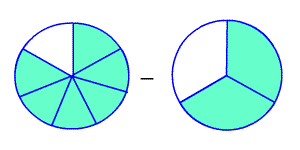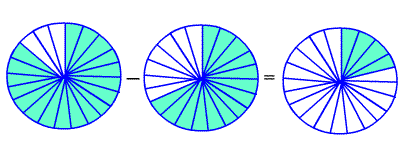# Subtracting Fractions with Unlike Denominators

If the denominators are not the same, then you have to use equivalent fractions which do have a common denominator . To do this, you need to find the least common multiple (LCM) of the two denominators.

To subtract fractions with unlike denominators, rename the fractions with a common denominator. Then subtract and simplify.

For example, suppose you want to add:

$\frac{6}{7}-\frac{2}{3}$The LCM of $3$ and $11$ is $33$ . So, we need to find fractions equivalent to $\frac{6}{7}$ and $\frac{2}{3}$ which have $21$ in the denominator. Multiply the numerator and denominator of $\frac{6}{7}$ by $3$ , and multiply the numerator and denominator of $\frac{2}{3}$ by $7$ .

$\frac{6\text{\hspace{0.17em}}×\text{\hspace{0.17em}}3}{7\text{\hspace{0.17em}}×\text{\hspace{0.17em}}3}-\frac{2\text{\hspace{0.17em}}×\text{\hspace{0.17em}}7}{3\text{\hspace{0.17em}}×\text{\hspace{0.17em}}7}=\frac{18}{21}-\frac{14}{21}$

Now we have like denominators, and we can subtract as described above.

$=\frac{4}{21}$